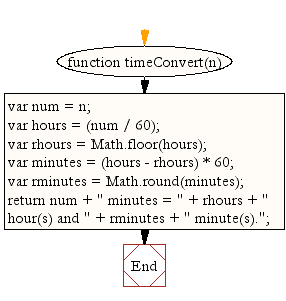# JavaScript: Return the number of minutes in hours and minutes

## JavaScript Datetime: Exercise-13 with Solution

Write a JavaScript function that returns the number of minutes in hours and minutes.

Test Data :
console.log(timeConvert(200));
Output :
"200 minutes = 3 hour(s) and 20 minute(s)."

Sample Solution:-

HTML Code:

``````<!DOCTYPE html>
<html>
<meta charset="utf-8">
<title>Return the number of minutes in hours and minutes.</title>
<body>

</body>
</html>
```
```

JavaScript Code:

``````function timeConvert(n) {
var num = n;
var hours = (num / 60);
var rhours = Math.floor(hours);
var minutes = (hours - rhours) * 60;
var rminutes = Math.round(minutes);
return num + " minutes = " + rhours + " hour(s) and " + rminutes + " minute(s).";
}

console.log(timeConvert(200));
```
```

Sample Output:

```200 minutes = 3 hour(s) and 20 minute(s).
```

Flowchart:Live Demo:

See the Pen JavaScript - Return the number of minutes in hours and minutes-date-ex- 13 by w3resource (@w3resource) on CodePen.

Improve this sample solution and post your code through Disqus

What is the difficulty level of this exercise?

Test your Programming skills with w3resource's quiz.

﻿

## JavaScript: Tips of the Day

Object.freeze

```const box = { p: 10, q: 20 };

Object.freeze(box);

const shape = box;
shape.p = 100;

console.log(shape);
```

Object.freeze makes it impossible to add, remove, or modify properties of an object (unless the property's value is another object).
When we create the variable shape and set it equal to the frozen object box, shape also refers to a frozen object. You can check whether an object is frozen by using Object.isFrozen. In this case, Object.isFrozen(shape) returns true, since the variable shape has a reference to a frozen object.
Since shape is frozen, and since the value of p is not an object, we cannot modify the property p. p is still equal to 10, and { p: 10, q: 20 } gets logged.

Ref: https://bit.ly/3jFRBje

We are closing our Disqus commenting system for some maintenanace issues. You may write to us at reach[at]yahoo[dot]com or visit us at Facebook